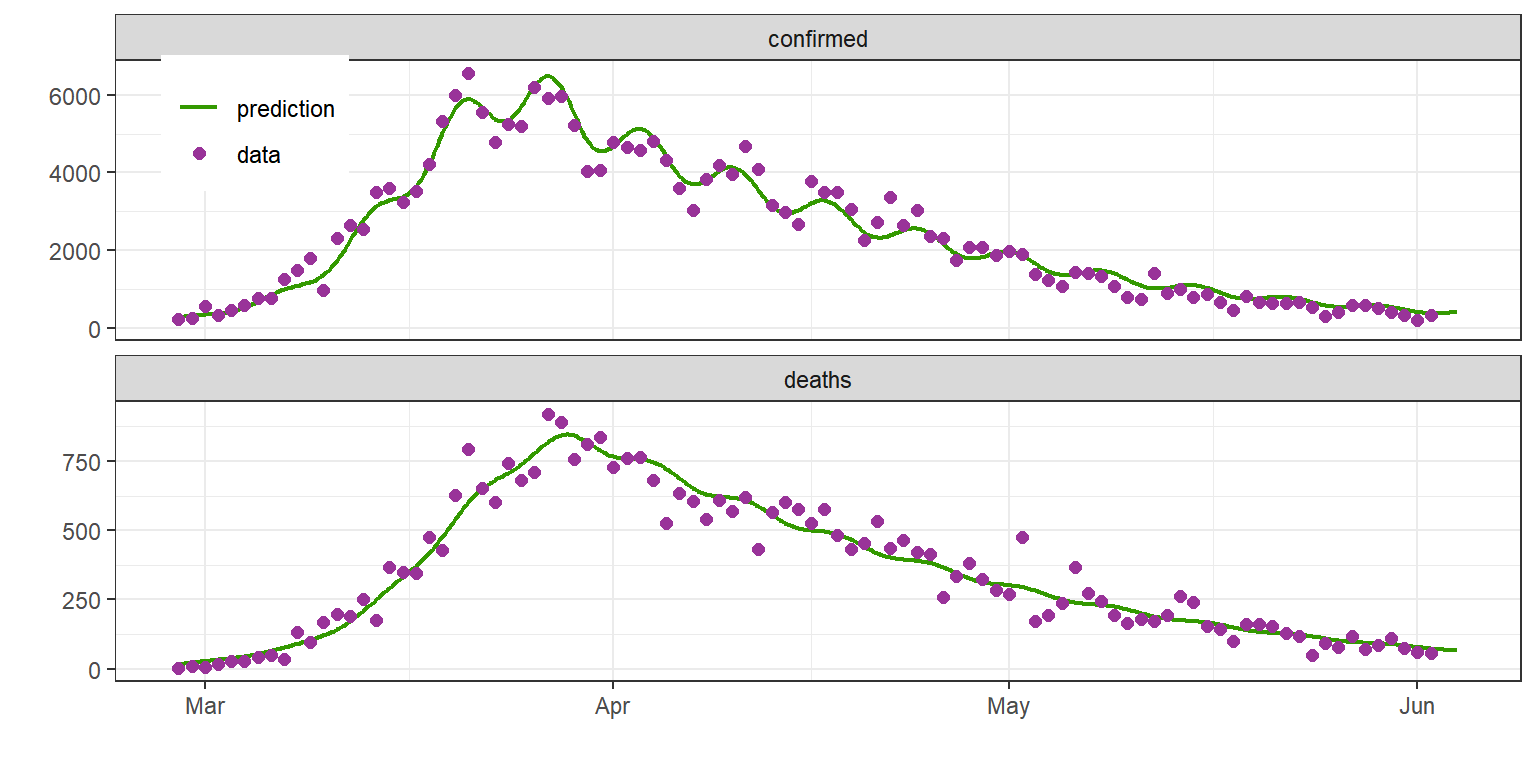$$\newcommand{\esp}{\mathbb{E}\left(#1\right)} \newcommand{\var}{\mbox{Var}\left(#1\right)} \newcommand{\deriv}{\dot{#1}(t)} \newcommand{\prob}{ \mathbb{P}\!(#1)} \newcommand{\eqdef}{\mathop{=}\limits^{\mathrm{def}}} \newcommand{\by}{\boldsymbol{y}} \newcommand{\bc}{\boldsymbol{c}} \newcommand{\bpsi}{\boldsymbol{\psi}} \def\pmacro{\texttt{p}} \def\like{{\cal L}} \def\llike{{\cal LL}} \def\logit{{\rm logit}} \def\probit{{\rm probit}} \def\one{{\rm 1\!I}} \def\iid{\mathop{\sim}_{\rm i.i.d.}} \def\simh0{\mathop{\sim}_{H_0}} \def\df{\texttt{df}} \def\res{e} \def\xomega{x} \newcommand{\argmin}{{\rm arg}\min_{#1}} \newcommand{\argmax}{{\rm arg}\max_{#1}} \newcommand{\Rset}{\mbox{\mathbb{R}}} \def\param{\theta} \def\setparam{\Theta} \def\xnew{x_{\rm new}} \def\fnew{f_{\rm new}} \def\ynew{y_{\rm new}} \def\nnew{n_{\rm new}} \def\enew{e_{\rm new}} \def\Xnew{X_{\rm new}} \def\hfnew{\widehat{\fnew}} \def\degree{m} \def\nbeta{d} \newcommand{\limite}{\mathop{\longrightarrow}\limits_{#1}} \def\ka{k{a}} \def\ska{k{\scriptscriptstyle a}} \def\kel{k{e}} \def\skel{k{\scriptscriptstyle e}} \def\cl{C{\small l}} \def\Tlag{T\hspace{-0.1em}{lag}} \def\sTlag{T\hspace{-0.07em}{\scriptscriptstyle lag}} \def\Tk{T\hspace{-0.1em}{k0}} \def\sTk{T\hspace{-0.07em}{\scriptscriptstyle k0}} \def\thalf{t{1/2}} \newcommand{\Dphi}{\partial_\pphi #1} \def\asigma{a} \def\pphi{\psi} \newcommand{\stheta}{{\theta^\star}} \newcommand{\htheta}{{\widehat{\theta}}}$$

Abstract

We propose to build a SIR-type model for the Covid-19 data provided by the Johns-Hopkins University. The model is adapted in order to fit the data for a given country. In particular, the model integrates a time-dependent transmission rate, whose variations can be thought to be related to the health measures taken by the country in question.

Our study focuses on what happened between early March and early June. Indeed, this period corresponds to what can be called the “first wave” in sevral European countries.

We have chosen to cover this period because various parameters related to the dynamics of the pandemic ( lethality rate, number of tests performed, infection rate,…) seem to have changed significantly afterwards.

Even if the proposed model adjusts the data very well, it is important to stress that such a model does not claim to be able to predict the evolution of the epidemic in each country. It merely proposes a possible scenario in the relatively short term, assuming a certain stability of conditions.

Note that an interactive application allows to visualize the data and the fitted model for several countries.

# 1 The data

library(covidix)
library(mlxR)
library(reshape2)
library(gridExtra)
library(dplyr)
library(tidyr)
library(ggplot2)
theme_set(theme_bw())

Let us start by reading the data provided by the Johns-Hopkins University and selecting some countries of interest

file0="covid19.csv"
rwCovid(file.out=file0)

# -- selected countries among 289 countries
list.country <- c("Italy", "Spain", "France", "Germany", "Netherlands", "Switzerland", "United Kingdom",  "US", "Brasil" )
d1 <- filterCovid(file.in=file0, country=list.country)

The data provided by the Johns-Hopkins University are, for each country:

1. the total number of confirmed cases $$(w_j \ ; j=1,2,\ldots)$$,
plotCovid(data=d1)[]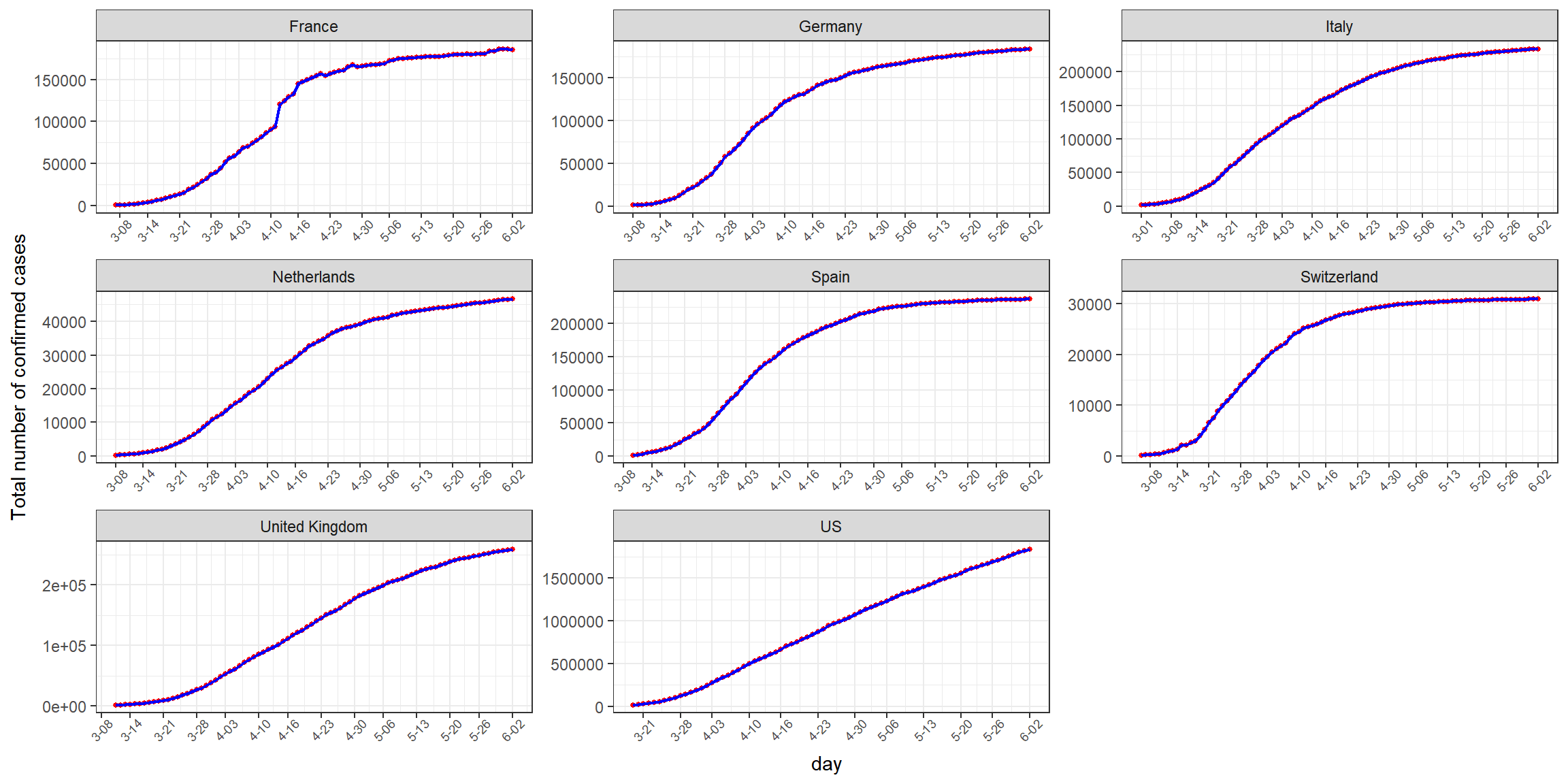1. the total number of deaths $$(d_j \ ; j=1,2,\ldots)$$
plotCovid(data=d1)[]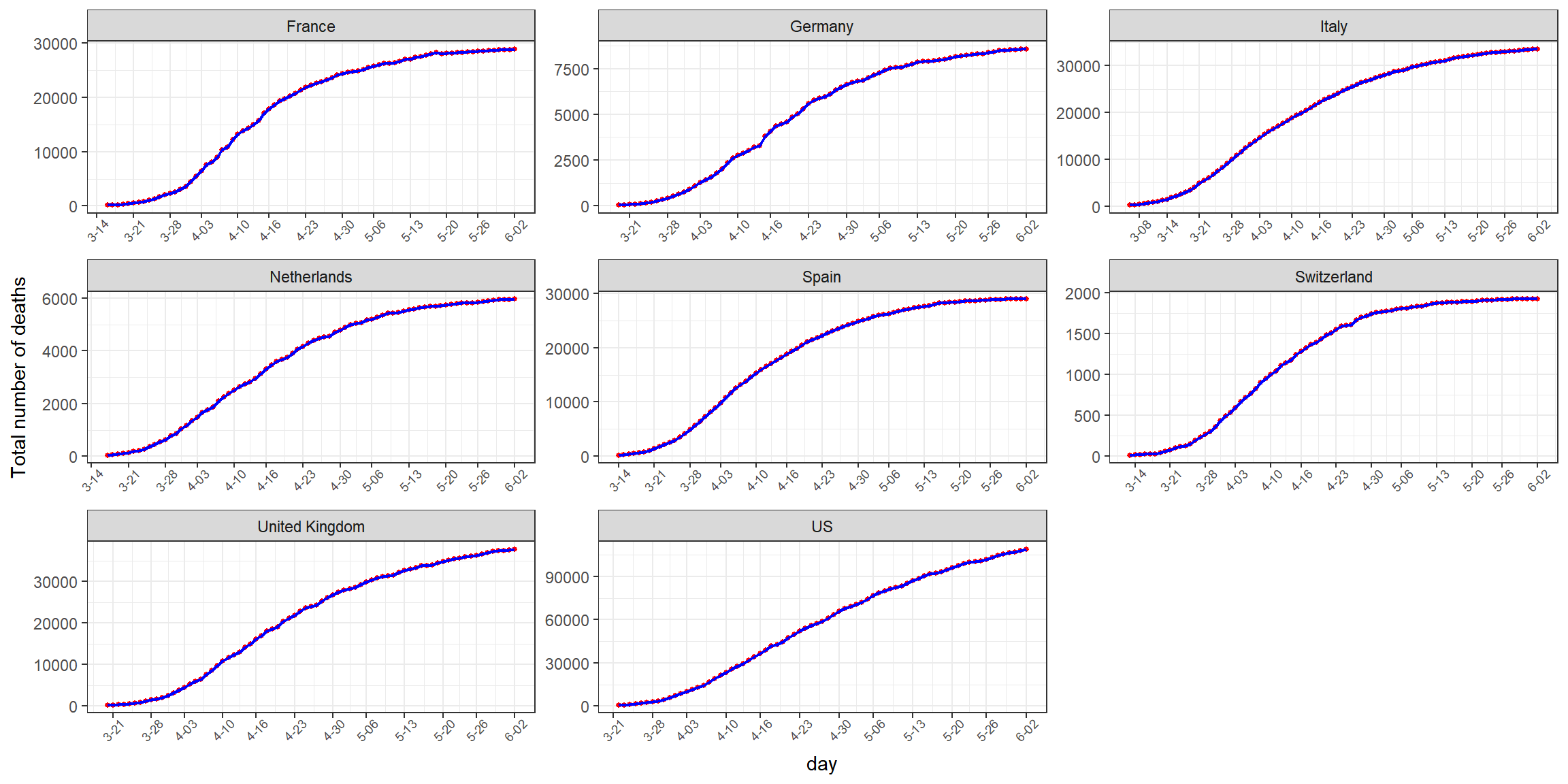We aim to build a model capable of describing this data.

# 2 A SIR model for this data

The model describes the dynamics of 4 subpopulations of individuals: \begin{aligned} \dot{S}(t) &= -\beta \frac{S(t)}{N}I(t) \\ \dot{I}(t) &= \beta \frac{S(t)}{N}I(t) - \mu I(t) -\nu I(t) \\ \dot{R}(t) &= \mu I(t) \\ \dot{D}(t) &= \nu I(t) \end{aligned} Here, $$S(t)$$, $$I(t)$$, $$R(t)$$ and $$D(t)$$ are, respectively, the numbers of susceptible, infectious, recovered and deceased individuals at time $$t$$.

We will make the following assumptions:

1. the number of infectious individuas in the population is negligible in front of the population size. We therefore consider that $$S(t)$$ can be replaced by $$N$$,

2. the transmission rate $$\beta$$ may change over time.

According to these hypotheses, the system writes \begin{aligned} \dot{I}(t) &= \beta(t) I(t) - \mu I(t) -\nu I(t) \\ \dot{R}(t) &= \mu I(t) \\ \dot{D}(t) &= \nu I(t) \end{aligned}

The next step will now be to link this model to the available data.

First, let $$W(t)$$ be the total number of infected cases at time $$t$$ (i.e. the total number of people who have been infected between the start of the epidemy and time $$t$$). Then, accoding to the model, $\dot{W}(t) = \beta(t) \, I(t)$

We should stress that not all the infected cases are recorded. We will assume that only a fraction $$\alpha$$ of the infected people are confirmed, i.e. are counted in the JHU records. Then, the total number of confirmed cases at the end of day $$j$$ is $$w_j=W_c(t_j)$$ where \begin{aligned} {W}_c(t) &= \alpha \, {W}(t) \end{aligned} Setting $$I_c(t) =\alpha I(t)$$, the model then becomes

\begin{aligned} \dot{I}_c(t) &= \beta(t) \, I_c(t) - \mu \, I_c(t) -\nu \, I_c(t) \\ \dot{W_c}(t) &= \beta(t) \, I_c(t) \\ \dot{D}(t) &= \frac{\nu}{\alpha} \, I_c(t) \end{aligned}

Next, a visual examination of the data reveals a time lag between the curve of confirmed cases and that of deaths. In the case of Italy, for example, a time lag of 4 days and a change of scale ($$r=\approx 0.14)$$ allows a almost perfect’’ superposition of the two series.

d <- filterCovid(file.in=file0, country="Italy", nc.min=200, nd.min=5)
r <- corfit(data=d, predict=F)
print(r$coef) ## shift scale ## 4.000 0.143 print(r$plot)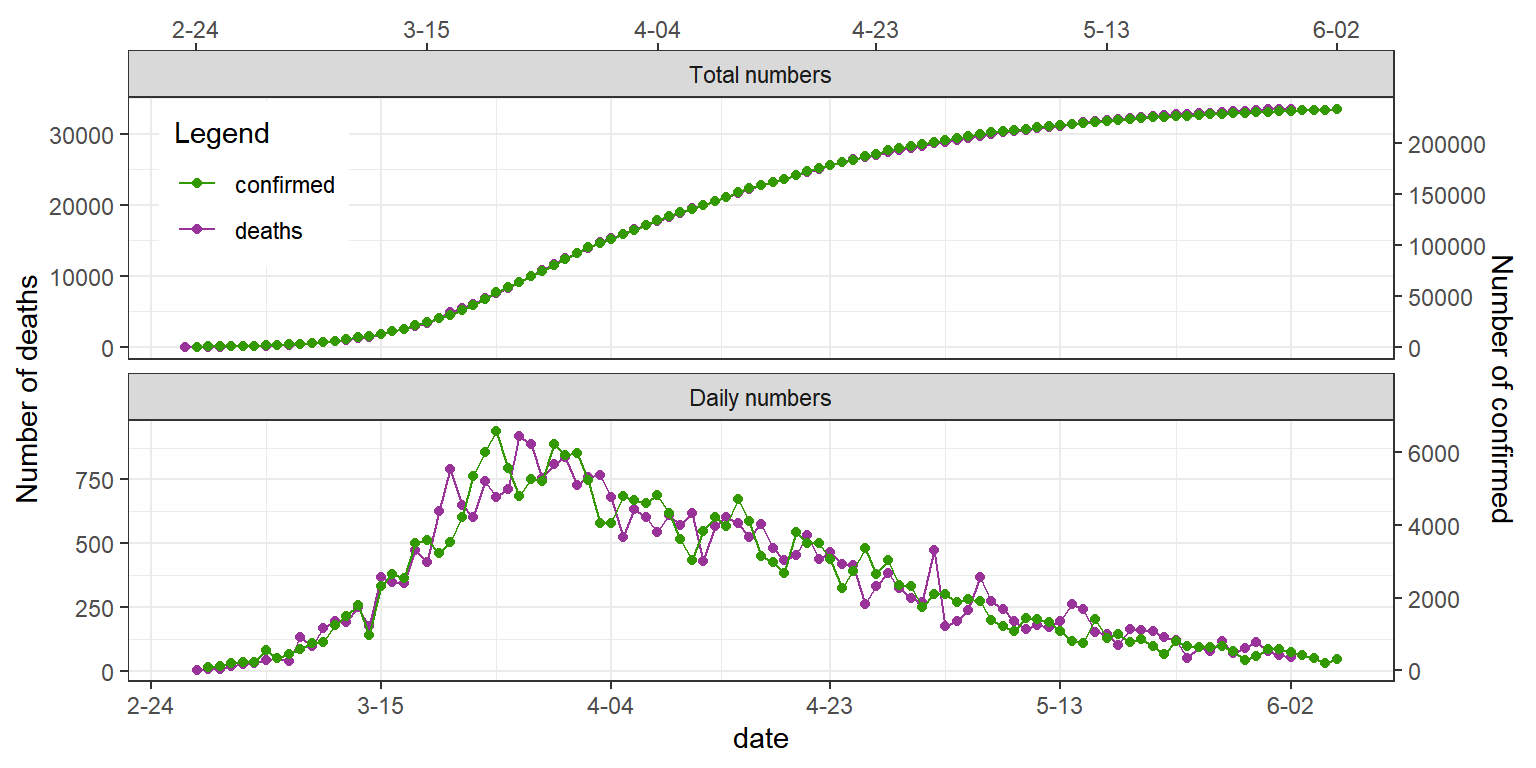We will therefore add an additional compartment $$L$$ in the model, which represents the stock of individuals who will die (because they were infected), but who don’t infect anybody (probably because they’re isolated).

Our final model writes: \begin{aligned} \dot{I}_c(t) &= \beta(t) \, I_c(t) - \mu \, I_c(t) -\nu \, I_c(t) \\ \dot{W_c}(t) &= \beta(t) \, I_c(t) \\ \dot{L}(t) &= \frac{\nu}{\alpha} \, I_c(t) - \lambda \, L(t) \\ \dot{D}(t) &= \lambda \, L(t) \end{aligned}

The dynamics of the infectious class defined by this model can be summarized by the effective reproduction number $R_{\rm eff}(t) = \frac{\beta(t)}{\mu + \nu}$ This ratio represents the expected number of secondary infections from a single infection. As the number of infected persons is supposed to be small compared to the number of susceptible persons, it can be noted here that $$R_{\rm eff}(t) \approx R_0(t)$$ where $$R_0(t)$$ is the basic reproduction number, i.e. the average number of secondary infections produced by a typical case of an infection in a population where everyone is susceptible.

In particular, $$R_{\rm eff}(t) = 1$$ means that $$\dot{I}_c(t)=\dot{I}(t)=0$$, which means that the daily number of newly infected individuals remains constant over time, or equivalently, that the total number of (confirmed) cases increases linearly.

# 3 Fitting the model to the data

## 3.1 Parameter estimation and model selection

Here, fitting the model to the data of a given country means

1. to select a model for the transmission rate function $$\beta$$,
2. to estimate the parameters of the model

Concerning the transmision rate function $$\beta$$, we will consider a piecewise linear continuous function.

More precisely, we assume that there exists a sequence of change points $$(\tau_1, \tau_2, \ldots, \tau_K)$$ such that $\beta(t) = \beta_0 + a\,t + \sum_{k=1}^{K} h_k \,( t - \tau_k) \times \one \{t\geq \tau_k \}$ and where $$a + h_1 + h_2 + \ldots + h_k$$ is the slope in segment $$k$$.

For a given number $$K$$ of segments, we need to estimate the parameters of the model and, possibly, the initial conditions of the system if we don’t start at $$t=0$$. Let $\theta = (\alpha, \beta_0, a, h_1, \ldots, h_{K-1}, \gamma_ \delta, \lambda, I_{c,0}, W_{c,0}, L_0, D_0)$

For identifiability reason, $$\alpha$$ cannot be estimated from the data. We arbitrarily set $$\alpha=1$$.

Imagine that we have data from day 1 to day $$J$$. Then, we look for the value of $$\theta$$ that gives the best fit’’ of the model, not only for the sequences of total counts $$(w_j , 1 \leq j \leq J)$$ and $$(d_j , 1 \leq j \leq J)$$, but also for the sequences of daily counts $$(w_j - w_{j-1} , 2 \leq j \leq J)$$ and $$((d_j - d_{j-1} , 2 \leq j \leq J)$$.

Let $$(\hat{w}_j(\theta), j=1,2,\ldots, J)$$ and $$(\hat{d}_j(\theta), j=1,2,\ldots, J)$$ be the sequences of total numbers of confirmed cases and total numbers of deaths predicted by the model for a given vector of parameters $$\theta$$.

Then, the vector of estimated parameters $$\hat{\theta}_K$$ minimizes the obective function $U(\theta) = c_1 \log(\hat \sigma_1^2(\theta)) + c_2 \log(\hat \sigma_2^2(\theta)) + c_3 \log(\hat \sigma_3^2(\theta)) + c_4 \log(\hat \sigma_4^2(\theta))$ where \begin{aligned} \hat \sigma_1^2(\theta) &= \frac{1}{J}\sum_{j=1}^J (w_j - \hat{w}_j(\theta))^2 \\ \hat \sigma_2^2(\theta) &= \frac{1}{J}\sum_{j=1}^J \left((w_j - w_{j-1}) - (\hat{w}_j(\theta) - \hat{w}_{j-1}(\theta))\right)^2 \\ \hat \sigma_3^2(\theta) &= \frac{1}{J}\sum_{j=1}^J (d_j - \hat{d}_j(\theta))^2 \\ \hat \sigma_4^2(\theta) &= \frac{1}{J}\sum_{j=1}^J \left((d_j - d_{j-1}) - (\hat{d}_j(\theta) - \hat{d}_{j-1}(\theta))\right)^2 \end{aligned} are the estimated variances of the four series and where $$c_1$$, $$c_2$$, $$c_3$$ and $$c_4$$ are relative weights given to each of these four criteria. By default, $$c_1=c_2=c_3=c_4=1$$.

The selected number of segments $$K$$ minimizes the Bayesian Information Criterion (BIC)

$BIC(K) = U(\hat{\theta}_K) + \log(4J)\times(2K-1)$

## 3.2 Including a weekly periodic component

Let us see, for example, the results obtained with Italy:

pl <- fitPlot(file="../shiny/data_June02/Italy.RData", plot.pred=F)
print(pl[])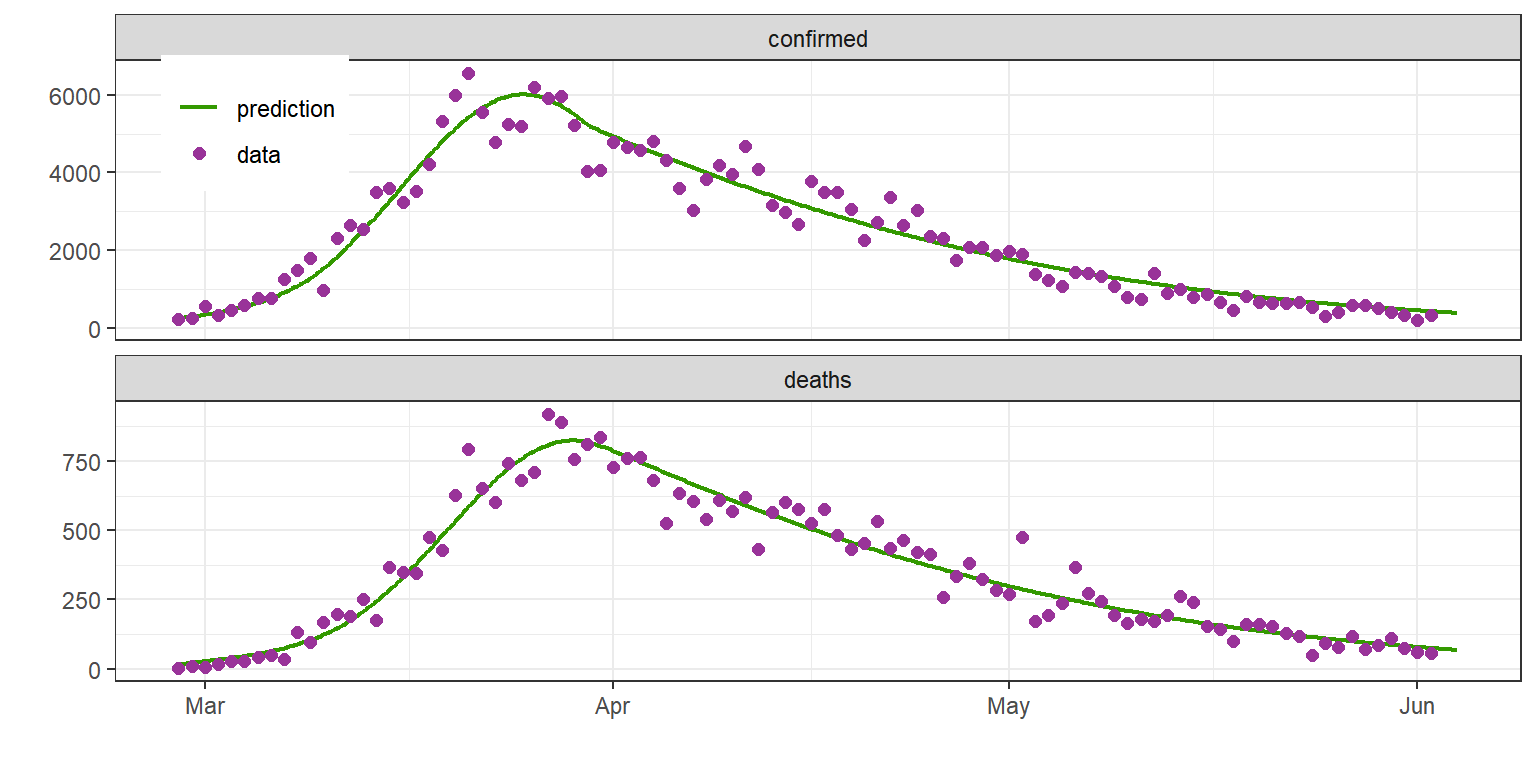The fit looks really nice… But let’s take a look at the residues

load("../shiny/data_June02/Italy.RData")
d$e0 <- d$value - d\$pred0
ggplot(data=subset(d, variable=='y'), aes(date, e0)) + geom_line() + geom_point() + facet_wrap(~type, ncol=1, scale="free_y")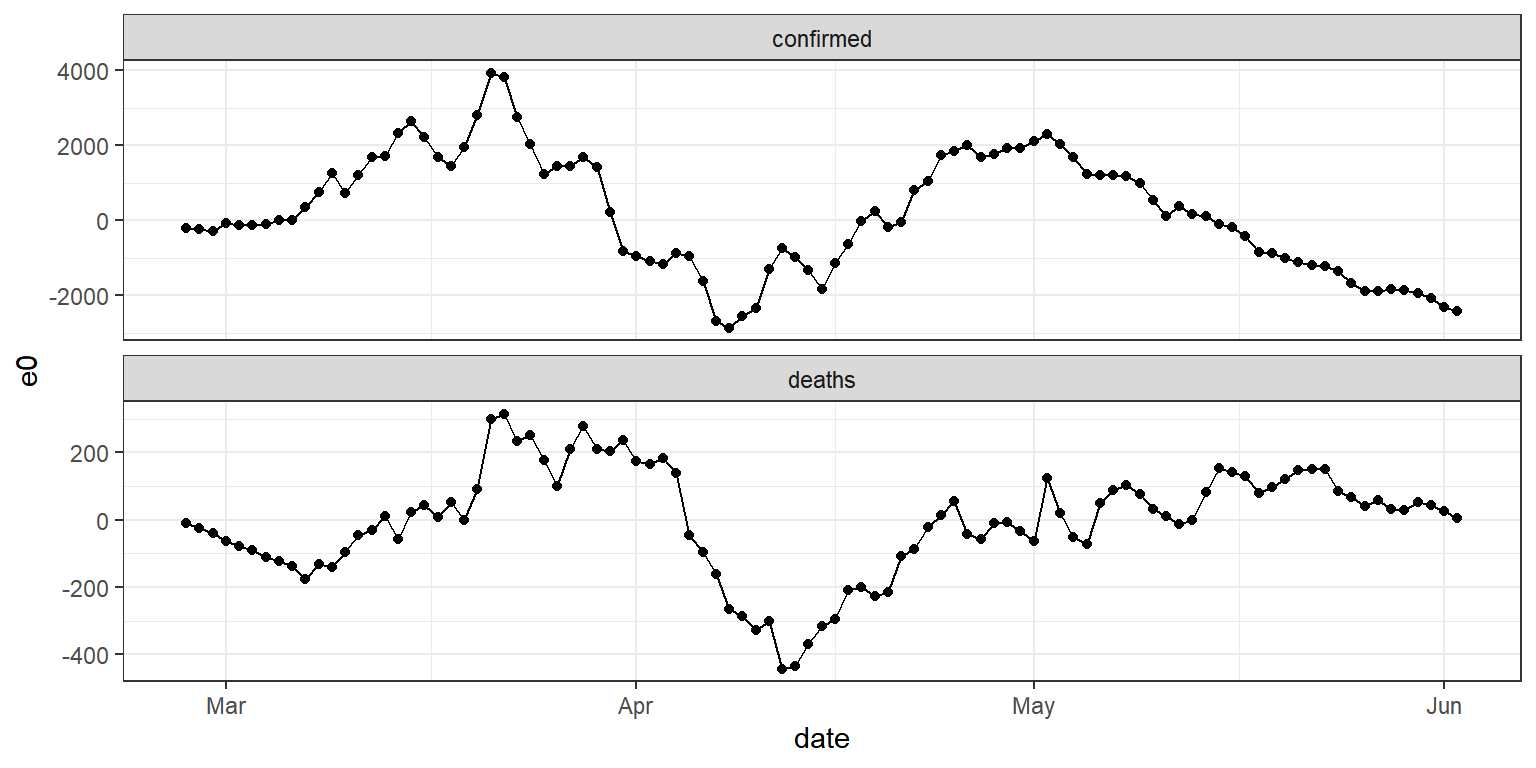The residues clearly exhibit a periodic component, with a 7-day period. In other words, it appears that the reported numbers fluctuate depending on the day of the week.

Note that it is not the true numbers that fluctuate, but the reported numbers. Then, the observation model should include this weekly periodic component.

\begin{aligned} w_j &= W_c(t_j)\left(1 + A \cos(\frac{2 \pi}{7} t_j + \phi) + e_{w_j}\right) \\ d_j &= D_c(t_j) \left(1 +B \cos(\frac{2 \pi}{7} t_j + \phi) + e_{d,j} \right) \end{aligned}

Additional parameters $$A$$, $$B$$ and $$\phi$$ are estimated simultaneously with the other set of parameters.

Here are the new adjustments obtained with this new model:

pl <- fitPlot(file="../shiny/data_June02/Italy.RData")
print(pl[])